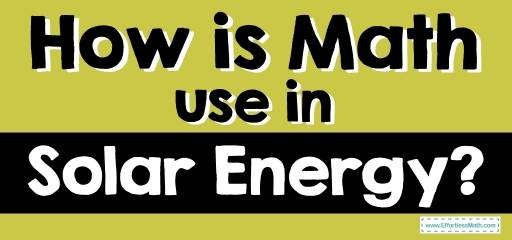# How Is Math Use in Solar Energy?Solar technology changes sunlight to electrical energy because the energy comes directly from the sun. Although solar energy has been in use for thousands of years, people are slowly starting to revert.

The cost of installing solar panels varies based on key factors: location, size of the home, and roof size. Other factors such as power levels, placement, and sunlight exposure are all valid questions.

Suppose you’re interested in buying solar panels for your three-bedroom home. You might be wondering how many solar panels to get for 2000 sq ft and how to calculate solar energy. These are all common questions for first-time solar panel buyers or anyone interested in learning about solar power calculations.

However, the thought of calculating solar energy could sound highly intimidating. This is because there are so many different types of terms used to measure energy. But never fear! This article will identify how math is used in solar energy.

How to calculate solar energy output is a question that homeowners must consider if they are interested in purchasing solar panels.

• The average house needs 20 to 25 solar panels to cover 100% of electricity.
• Other factors are geographical location, rate power of panel, the efficiency of the solar panels, and energy consumption habits.
• The more panels need the more pricey the overall cost.

So, an excellent place to start is by brushing up on units of measurement. Not only is the terminology vital to solar panels. Look around your house and specifically look at any device that uses electricity. You will see some of these terms on the back of your devices. So here are units of measurement terminology:

Electricity is a form of energy created from positive and negative protons.

Amp is a standard unit used to determine how fast a current is flowing.

Volt measures the force of electricity through a circuit by conducting looping and enabling the device to work.

Watts is a unit of power that equals one joule per second. Used to quantify the rate of energy

Ohms is a unit of electric resistance. The amount of resistance necessary to charge a current to flow when a volt is applied.

## Bottle filling Station Example:

You’re thirsty, and you need a drink of water from the bottle filling station. For this process to work, water has to come out and an electrical pressure to force it out the pipes and into the water bottle.

Electrical pressure is called voltage one has a positive charge and a negative charge. Electrons flow from the negative end to whichever direction you want the energy to flow into the positive end in a battery.

So, Amps and Volts together tell you how much current is flowing and how much pressure is applied.

## How to calculate Solar Energy with Math?

The terms above work together to find any electric applications volt, amps, and watts. Ohm’s law is a formula relationship between voltage, current, resistance, and electric circuits and how they recharge: Ohm’s Law: (E = IR). The formula is only essential when measuring the volts, wires, and distances between batteries (solar panels, charge controller, etc.)

Watts = Volts (V 0 x Amps (A)

Breakdown of calculations

Convert: Watts to Amps: Amps = Watts / Volts

= 12 Watts / 12 Volts = 1 Amp

Convert: Amps to Watts:      1 Amp X 12  Volt = 12 Watts

= 120 Watts / 10 Amps = 12 Volts

Convert: Volts to Watts:         Watts = Amps x Volt

= 12 Amps x 12 Volts

= 144 Watts

Use this formula to investigate the size of solar panels, batteries, storage, charge controller, and the necessary converters for calculations. Not to mention, observe the weather more—necessary to record time between sunny days and cloudy days.

As previously stated, watts and amps are multiplied together and measured by time for any given voltage. Thus, watt (hours) is used to analyze the inward flow of solar energy. The energy then projects off the energy devices that are charging.

Finally, Amps used stored energy: It’s all a cycle.

## The calculations for solar panels in the home are the same:

Instead, you’re just looking for the Annual Electricity Usage. The total amount of electricity used in a home tv, smartphone charging station, fridge, washer, dryer,  and dishwasher, all of that is total. Average America used 10,649 kWh per year, according to U.S. Energy Information Administration (EIA).

Solar Panel Usage or panel power is the electrical output of a solar panel. Wattage (W) ranges from 150 to 370 depending on the size and quality of the panel.

The production Ratio varies on the graphic location. In the United States, the average production ratio is 1.3 to 1.6.

All the terms are identified: Average Electrical Usage  (10,649)

Solar panel usage.                (370)

Production Ratio                 (1.3 0r 1.6)

Number of panels = system size/production ratio/ panels wattage

Simply plug in the numbers on a calculator.

10,649 kW / 1.3 or 1.6 / 370 W

The answer is 17 to 22 panels.

## Summary

Even if you never use these calculations again, understanding how to carry out this formula is beneficial. These calculations can also show how much electricity you’re using if you have many panels. Plus, if you understand how to calculate solar, you can figure out how to calculate regular energy.

Even if you do not buy solar panels, these calculations are the gateway to learning about energy and saving on energy.

Americans across the country use about 10,649 energy. Exactly how much of that energy is solar-powered or fossil fuels? That is a topic for another day.

### What people say about "How Is Math Use in Solar Energy? - Effortless Math: We Help Students Learn to LOVE Mathematics"?

No one replied yet.

X
30% OFF

Limited time only!

Save Over 30%

SAVE \$5

It was \$16.99 now it is \$11.99